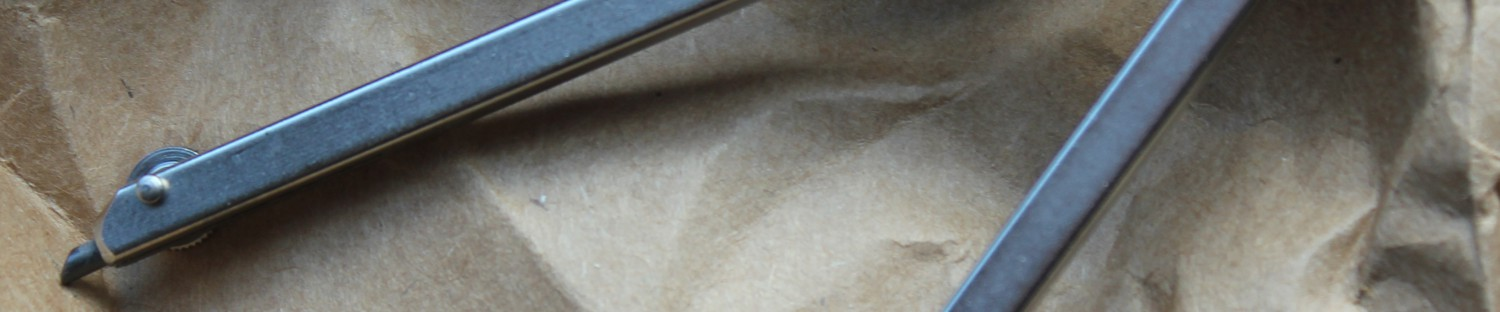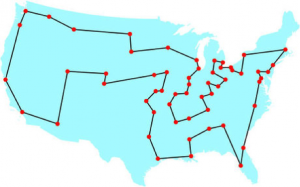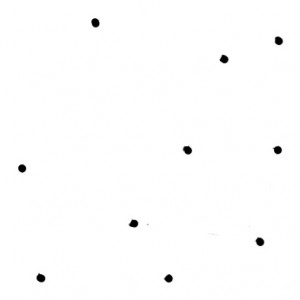# The Geometric Viewpoint

geometric and topological excursions by and for undergraduates## Delauney Triangulations and the Traveling Salesman

The Traveling Salesman and Delauney Triangulation

By Aaron Liu

To quote Death of a Salesman, “A salesman is got to dream, boy”, it is easy to understand the struggles that come with being a traveling salesman. I like to think that the main character was dreaming of a quick way to approximate a path from his starting location to every city and back. I know that that might not have been his exact dream, but if I were a salesman I would dream of a solution to this problem.

It is hard to imagine the struggles of the traveling salesman, but if you take a quick look at the picture below, you will understand why a salesman must dream. Must I remind you that the distance from Boston to California as shown on the map is approximately: 3,109.3 miles. That’s about 5003.9333 kilometers for all of my European readers.Currently there is no effect method of solving this problem for all situations. There are no known algorithms that will work 100% of the time and a lot of people have come to accept that fact (not me). But, there are ways to approximate solutions to the traveling salesman problem and one way involves topology. More importantly, I’m going to use the brilliant work of Boris Delauney and his triangulation in order to detail the approximations.

Triangulation Basics

I bet you are wondering how to create a Delauney Triangulation. Lucky for you I’m about to explain just that. Let’s start off with a simple graph in order to understand the basics.In order create a Delauney Triangulation we need to consider all of the circles around sets of 3 points. Generally, if we start on the outside we can work our way inward. These such circles are called circumcircles and the key aspect is the fact that no point on the graph is inside a circumcircle. This may sound very complex and hard to achieve and that may be true for huge graphs, but for our example the Delauney Triangulation is actually quite simple.It is easy to picture if you isolate the circles. Notice how the circumcircles overlap, but no point is inside of a circumcircle. Above all of the circles created is the triangulation that we wanted. The triangles are created by the circumcircles. Every point is connected with an edge to points that it shares a common circle with. It is important to note that if there is ever a circle with four points on it, the Delauney Triangulation will not exist. Conceptually this is makes sense because if that were allowed, we might have to call it a Delauney Polygonation. Sorry for that terrible joke.

In any event, the circumcircles allow us to create the triangulation and also give the triangulation some key features. But, first here are some important concepts.

Important Concepts:

A simplex is a dimensional piece of the whole. A 0-simplex would be a point, a 1-simplex would be a line segment, a 2-simplex would be a triangle, a 3 simplex would be a tetrahedron and so on.

Also the convex hull is the points such that all other points can be contained within those points. In the graph below the points P0, P1, P3, P10, P12 are the convex hull.Now that you are enlightened with those key definitions, here are some key features of the triangulation:

• The union of all the simplicies is the convex hull of the points
• The triangulation maximizes the minimum angle of the triangles

This second characteristic means that the smallest angle of a triangle in the triangulation tends to be high. The triangulation rarely has scalene triangles because that would mean that two of the points were close together and the other was far away. This rarely occurs because if that were the case then the circle would likely be much bigger and has a higher chance of containing a point on the interior.

Delauney triangulations are derived from Voronoi diagrams. A Voronoi diagram can be created by connected the centers of the circumcircles of a Delauney triangulation. If you want to know more about the diagrams, take a quick look at Vlad’s post.

If not, or you believe you have a strong grasp on the triangulation, continue reading about the traveling salesman.

Delauney Triangulation and the Traveling Salesman

After seeing the above triangulation, it is hard to obviously see how the triangulation can be used to find a shortest path. The importance of approximating the traveling salesman problem is the quickness and in this situation, the ability to find a working solution. In the case of a Delauney Triangulation we can use the fact that none of the points are inside any other triangle. This is important because that means the triangulation has edges between points that are the closest to each other. It also gives us enough edges that we can construct a fairly accurate approximation.

Delauney Triangulations simply approximate so there are a lot of different algorithms that can be used. Also, the algorithms tend to grow to many and many lines of code that are very difficult to understand. Thus, I will explain a few such algorithms.

Algorithm Basics

Constructing an algorithm to approximate the shortest path using Delauney Triangulations can be very difficult. However, a lot of the characteristics of the Delauney Triangulation are very relevant to creating a shortest path algorithm.

Consider the following Delauney Triangulation:Looking at this triangulation it is easy to see how an approximation to the shortest cycle could exist. The edges minimize the distance between the points and allow us to connect all points of the graph. Also, it is important to note that the amount of edges needed to be checked is much less than if there had been an edge between each vertex.

In order to find an approximation you can systematically check and eliminate unnecessary edges of the graph. The methods of doing this will vary greatly and will have to be very specific. The level of accuracy of the approximation will depend on how the edges are checked and removed.

Algorithms

The easiest method of doing this would be a brute-force check of all of the possible paths and eliminate the edges that are not in it. This method can be time-consuming, but would still be comparatively fast and accurate.

Another method would be a variation of the brute-force, but would compare the edges used in the path to the length of the path. The algorithm would look for certain edges that are contained in longer paths and would eliminate them. The algorithm would continue to eliminate edges, which would decrease the amount of work needed to find an approximation.

There are so many different variations of an approximation algorithm because after all it is approximation. One last algorithm that might be useful is checking a random amount of random paths and simply returning the path that produced the smallest distance. This obviously is not as accurate as the other algorithms, but is nonetheless useful if a quick solution is needed.

These algorithms are similar to an algorithm implemented by Christine T. Phan. Her paper can be found here.

It is important to note that at the end of her paper she states her results. She said on average Delauney Triangulations contained about 99.28% of the edges in the true shortest path. Using her own algorithm, similar to the ones described above, she was able obtain a 76.1% accuracy with her approximations. Although that number is not incredibly high, the results show that there are algorithms that will accurately approximate solutions to the problem. Perhaps, you will one day develop such an algorithm.

Conclusion

It has been shown that although Delauney Triangulations do not necessarily contain the optimal path, the triangulations contain edges that are in the optimal path. Thus, the quick approximation is very valuable and non-time-consuming. Delauney Triangulations are constructed in a way that is ideal towards finding a shortest path. Below are some resources that you can read if you want to learn more about Delauney Triangulations and the Traveling Salesman Problem. I guess until you do some more research and look into solving the problem, a salesman will just continue to dream.

Aaron Liu was a student in Scott Taylor’s Fall 2014 Topology class.

References:

http://www.geom.uiuc.edu/~samuelp/del_project.html

http://en.wikipedia.org/wiki/Delaunay_triangulation

http://oas.uco.edu/00/Papers/phan.htm

http://www.nytimes.com/interactive/2012/03/01/theater/201203-death-of-a-salesman-interactive.html

http://kiharalab.org/3d-surfer/v1.0/convex_hull.gif

This entry was posted in Uncategorized. Bookmark the permalink.